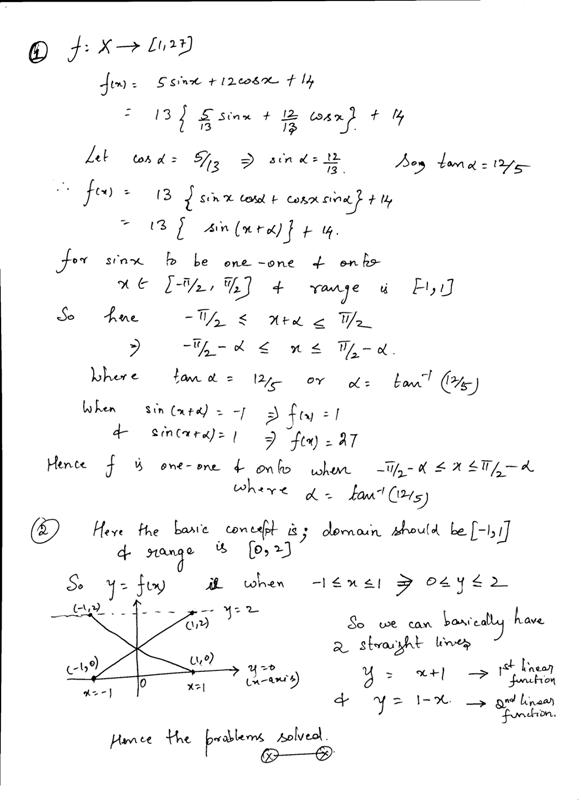# uestion 1 )         let f; X = [1,27] be a function  defined by f(x) = 5sinx +12cosx +14. find the set x so that set X so that f is both one one onto.ans:X = [-pi/2 - theta, pi/2 - theta] theta = tan-112/5question 2) find all the linear funtions defined on R such that they map [-1,1] onto  [0,2] and h; R -{-1,0,1}  R defined by h(x) = f(x)/ g(x)

11 years ago

Hi Shiwani,Hope that helps.

All the best.

Regards,